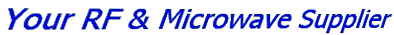# VCO Glossary - LTEQ MICROWAVEGo to content

## VCO Glossary

Active Components > VCO
VCO Glossary

• Voltage Controlled Oscillator (VCO): Type of oscillator that is continuously tunable by the application of a control voltage.
• Frequency Tuning performance: The characteristic frequency vs. tuning voltage curve generated by a VCO when tuned from the minimum to the maximum tuning voltage extremes. Typically presented in a graph format as Frequency vs. Voltage.
• Tuning Sensitivity or Modulation Sensitivity: The slope of the characteristic frequency tuning curve at a given tuning voltage (MHz/V).
• Monotonic Tuning: Continuous positive or negative change in the frequency tuning curve.
• Tuning Linearity: The amount of frequency deviation from a best fit straight line of the frequency vs. tuning voltage curve (MHz).
• Output Power Variation: The variation of the output power of the fundamental frequency when observed over the specified frequency range into a 50 ohm load at a fixed temperature (dB).
• Power Flatness: The variation of the output power from the average output power (dB).
• Output Power Variation with Temperature: The variation of the output power with respect to a change in the device's temperature (dB/C).
• Frequency Drift with Temperature: The variation of the output frequency with respect to a change in the devices temperature (MHz/C).
• Spurious Output (Non-Harmonic): Unwanted frequencies not harmonically related to the fundamental frequency (dBc).
• Harmonic Suppression: The level of harmonics (nfo) relative to the level of the fundamental frequency (dBc).
• Frequency Pushing: The variation of the output frequency due to a change in the supply voltage (MHz/V).
• Frequency Pulling: The variation of the output frequency due to a change in the output load conditions. Typically specified into a 12 dB Return Loss (MHz).
• 3 dB Modulation Bandwidth: The modulating frequency at which the frequency deviation decreases to 0.707 of its DC value. This is also a function of the modulating source impedance, which is typically 50 ohms.
• Phase Noise: The single side band phase noise in a 1 Hz bandwidth, measured relative to the carrier level at a specified offset frequency (dBc/Hz).
• VCO Input Capacitance: The total equivalent input capacitance seen at the tuning port of the VCO (pF).
• Microphonic: Spurious signals seen on the VCO output when the device is subjected to vibration or shock.
• Frequency Drop Outs: The sudden unexpected loss of frequency output while tuning across the frequency range.

Back to content